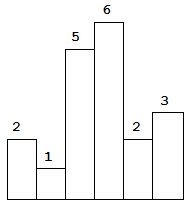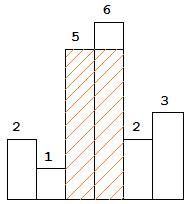# LeetCode 88. Merge Sorted Array

## Description

Merge Sorted Array

Given two sorted integer arrays nums1 and nums2, merge nums2 into nums1 as one sorted array.

Note:

• The number of elements initialized in nums1 and nums2 are m and n respectively.

• You may assume that nums1 has enough space (size that is greater or equal to m + n) to hold additional elements from nums2.

Example:

``````Input:
nums1 = [1,2,3,0,0,0], m = 3
nums2 = [2,5,6],       n = 3

Output: [1,2,2,3,5,6]
``````

`nums1``nums2`两个有序数组合并到`nums1`一个中，`nums1`已分配足够空间存放元素。

# LeetCode 87. Scramble String

## Description

Scramble String

Given a string s1, we may represent it as a binary tree by partitioning it to two non-empty substrings recursively.

Below is one possible representation of s1 = `"great"`:

``````    great
/    \
gr    eat
/ \    /  \
g   r  e   at
/ \
a   t
``````

To scramble the string, we may choose any non-leaf node and swap its two children.

# LeetCode 86. Partition List

## Description

Partition List

Given a linked list and a value x, partition it such that all nodes less than x come before nodes greater than or equal to x.

You should preserve the original relative order of the nodes in each of the two partitions.

Example:

``````Input: head = 1->4->3->2->5->2, x = 3
Output: 1->2->2->4->3->5
``````

# LeetCode 85. Maximal Rectangle

## Description

Maximal Rectangle

Given a 2D binary matrix filled with 0's and 1's, find the largest rectangle containing only 1's and return its area.

Example:

``````Input:
[
["1","0","1","0","0"],
["1","0","1","1","1"],
["1","1","1","1","1"],
["1","0","0","1","0"]
]
Output: 6
``````

# LeetCode 84. Largest Rectangle in Histogram

## Description

Largest Rectangle in Histogram

Given n non-negative integers representing the histogram's bar height where the width of each bar is 1, find the area of largest rectangle in the histogram.Above is a histogram where width of each bar is `1`, given height = `[2,1,5,6,2,3]`.The largest rectangle is shown in the shaded area, which has area = `10` unit.

# LeetCode 82. Remove Duplicates From Sorted List II

## Description

Remove Duplicates from Sorted List II

Given a sorted linked list, delete all nodes that have duplicate numbers, leaving only distinct numbers from the original list.

Example 1:

``````Input: 1->2->3->3->4->4->5
Output: 1->2->5
``````

Example 2:

``````Input: 1->1->1->2->3
Output: 2->3
``````

# LeetCode 81. Search in Rotated Sorted Array II

## Description

Search in Rotated Sorted Array II

Suppose an array sorted in ascending order is rotated at some pivot unknown to you beforehand.

(i.e., `[0,0,1,2,2,5,6]` might become `[2,5,6,0,0,1,2]`).

You are given a target value to search. If found in the array return `true`, otherwise return `false`.

Example 1:

``````Input: nums = [2,5,6,0,0,1,2], target = 0
Output: true
``````

Example 2:

``````Input: nums = [2,5,6,0,0,1,2], target = 3
Output: false
``````

• This is a follow up problem to Search in Rotated Sorted Array, where nums may contain duplicates.
• Would this affect the run-time complexity? How and why?

# LeetCode 80. Remove Duplicates from Sorted Array II

## Description

Remove Duplicates from Sorted Array II

Given a sorted array nums, remove the duplicates in-place such that duplicates appeared at most twice and return the new length.

Do not allocate extra space for another array, you must do this by modifying the input array in-place with O(1) extra memory.

Example 1:

``````Given nums = [1,1,1,2,2,3],

Your function should return length = 5, with the first five elements
of nums being 1, 1, 2, 2 and 3 respectively.

It doesn't matter what you leave beyond the returned length.
``````

Example 2:

``````Given nums = [0,0,1,1,1,1,2,3,3],

Your function should return length = 7, with the first seven elements
of nums being modified to 0, 0, 1, 1, 2, 3 and 3 respectively.

It doesn't matter what values are set beyond the returned length.
``````Programer

ChengDu·China